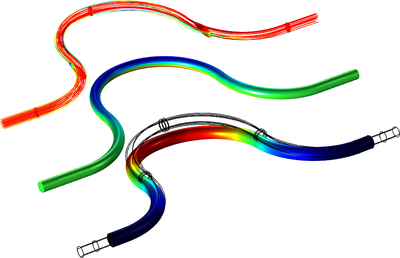# Galleria delle Applicazioni

## Coriolis Flowmeter: FSI Simulation in the Frequency Domain

Application ID: 51831

A Coriolis flowmeter, also known as a mass flowmeter or an inertial flowmeter, is used to measure the mass flow rate of a fluid traveling through it. It makes use of the fact that the fluid's inertia through an oscillating tube causes the tube to twist in proportion to the mass flow rate. Typically, the density and thereby the volumetric flow rate can also be assessed using the device.

This model shows how to simulate a generic Coriolis flowmeter with a curved geometry. When the fluid passes through the elastic structure (a curved duct), it interacts with the movement of the duct when vibrating. The phase difference between the deformation of two points on the duct is caused by the Coriolis effect and can be used to evaluate the mass flow rate through the system.

This is modeled in COMSOL Multiphysics using the Linearized Navier-Stokes, Frequency Domain interface coupled to the Solid Mechanics interface using the built-in multiphysics coupling. The background mean flow is modeled using the Turbulent Flow, SST interface. In this way, fluid-structure interaction (FSI) can be modeled efficiently in the frequency domain.

The model is based on an example from literature.

Learn more in the related blog post: " Modeling Aeroacoustics with the Linearized Navier-Stokes Equations ".This model example illustrates applications of this type that would nominally be built using the following products: